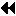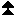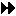## 4.14.1. Type Constructors with Explicit AddressesUp: New Datatype Manipulation Functions Next: Extent and Bounds of Datatypes Previous: New Datatype Manipulation Functions

The four functions below supplement the four corresponding type constructor functions from MPI-1. The new functions are synonymous with the old functions in C/C++, or on Fortran systems where default INTEGERs are address sized. (The old names are not available in C++.) In Fortran, these functions accept arguments of type INTEGER(KIND=MPI_ADDRESS_KIND), wherever arguments of type MPI_Aint are used in C. On Fortran 77 systems that do not support the Fortran 90 KIND notation, and where addresses are 64 bits whereas default INTEGERs are 32 bits, these arguments will be of type INTEGER*8. The old functions will continue to be provided for backward compatibility. However, users are encouraged to switch to the new functions, in both Fortran and C.

The new functions are listed below. The use of the old functions is deprecated.

MPI_TYPE_CREATE_HVECTOR( count, blocklength, stride, oldtype, newtype)
[ IN count] number of blocks (nonnegative integer)
[ IN blocklength] number of elements in each block (nonnegative integer)
[ IN stride] number of bytes between start of each block (integer)
[ IN oldtype] old datatype (handle)
[ OUT newtype] new datatype (handle)

int MPI_Type_create_hvector(int count, int blocklength, MPI_Aint stride, MPI_Datatype oldtype, MPI_Datatype *newtype)

MPI_TYPE_CREATE_HVECTOR(COUNT, BLOCKLENGTH, STIDE, OLDTYPE, NEWTYPE, IERROR)
INTEGER COUNT, BLOCKLENGTH, OLDTYPE, NEWTYPE, IERROR

MPI::Datatype MPI::Datatype::Create_hvector(int count, int blocklength, MPI::Aint stride) const

MPI_TYPE_CREATE_HINDEXED( count, array_of_blocklengths, array_of_displacements, oldtype, newtype)
[ IN count] number of blocks --- also number of entries in
array_of_displacements and array_of_blocklengths (integer)
[ IN array_of_blocklengths] number of elements in each block (array of nonnegative integers)
[ IN array_of_displacements] byte displacement of each block (array of integer)
[ IN oldtype] old datatype (handle)
[ OUT newtype] new datatype (handle)

int MPI_Type_create_hindexed(int count, int array_of_blocklengths[], MPI_Aint array_of_displacements[], MPI_Datatype oldtype, MPI_Datatype *newtype)

MPI_TYPE_CREATE_HINDEXED(COUNT, ARRAY_OF_BLOCKLENGTHS, ARRAY_OF_DISPLACEMENTS, OLDTYPE, NEWTYPE, IERROR)
INTEGER COUNT, ARRAY_OF_BLOCKLENGTHS(*), OLDTYPE, NEWTYPE, IERROR

MPI::Datatype MPI::Datatype::Create_hindexed(int count, const int array_of_blocklengths[], const MPI::Aint array_of_displacements[]) const

MPI_TYPE_CREATE_STRUCT(count, array_of_blocklengths, array_of_displacements, array_of_types, newtype)
[ IN count] number of blocks (integer) --- also number of entries in arrays array_of_types, array_of_displacements and array_of_blocklengths
[ IN array_of_blocklength] number of elements in each block (array of integer)
[ IN array_of_displacements] byte displacement of each block (array of integer)
[ IN array_of_types] type of elements in each block (array of handles to datatype objects)
[ OUT newtype] new datatype (handle)

int MPI_Type_create_struct(int count, int array_of_blocklengths[], MPI_Aint array_of_displacements[], MPI_Datatype array_of_types[], MPI_Datatype *newtype)

MPI_TYPE_CREATE_STRUCT(COUNT, ARRAY_OF_BLOCKLENGTHS, ARRAY_OF_DISPLACEMENTS, ARRAY_OF_TYPES, NEWTYPE, IERROR)
INTEGER COUNT, ARRAY_OF_BLOCKLENGTHS(*), ARRAY_OF_TYPES(*), NEWTYPE, IERROR

static MPI::Datatype MPI::Datatype::Create_struct(int count, const int array_of_blocklengths[], const MPI::Aint array_of_displacements[], const MPI::Datatype array_of_types[])

[ IN location] location in caller memory (choice)

<type> LOCATION(*)
INTEGER IERROR